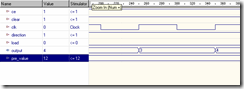Simulation of Synchronous and Asynchronous counters

Requirements:

• WARP
• Active HDL
• Cool Runner and Fitter CPLD Kit

Program for Asynchronous counter:
Library ieee;
Use ieee.std_logic_1164.all;
entity asyn_counter is
port (
clk: in BIT;
ce: in BIT;
clear: in BIT;
dir: in BIT;
p: in INTEGER range 0 to 255;
qd: out INTEGER range 0 to 255
);
end asyn_counter;
architecture asyn_counter of asyn_counter is
begin
variable count : integer range 0 to 255;
begin
if(clear = '0') then
count := 0;
elsif(load = '1' and clear = '1') then
if(ce = '1' and dir = '0') then
count := count + 0;
elsif(ce = '1' and dir = '1') then
count := count + 1;
end if;
end if;
qd <= count;
end process;
end asyn_counter;

Asynchronous counter

Synchronous Counter

Program for Synchronous Counter
library IEEE;
use IEEE.std_logic_1164.all;
entity syn_counter is
port (
clk: in STD_LOGIC;
ce: in STD_LOGIC;
clear: in STD_LOGIC;
direction: in STD_LOGIC;
pre_value: in INTEGER range 0 to 15;
output: out INTEGER range 0 to 15
);
end syn_counter;
architecture syn_counter of syn_counter is
begin
process(clk)
variable temp: integer range 0 to 15;
begin
if(rising_edge(clk)) then
if(clear = '0') then
temp := 0;
temp := pre_value;
elsif(ce='1' and direction = '0') then
temp := (temp -1) mod 16;
elsif(ce = '1' and direction = '1') then
temp := (temp +1) mod 16;
end if;
end if;
output <= temp;
end process;
end syn_counter;